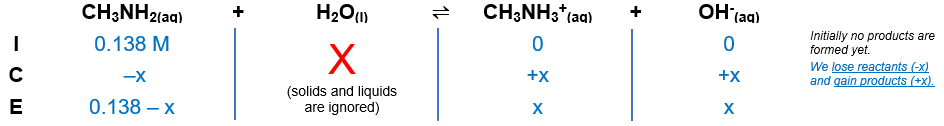# Problem: What is the molar solubility of Pb(OH)2 in a 0.138-M solution of CH3NH2?

###### FREE Expert Solution

CH3NH2 → weak base

Step 1: Calculate OH in the solutionKb of CH3NH2 = 4.4×10‒4

${\mathbf{K}}_{\mathbf{b}}\mathbf{=}\frac{\left[{\mathrm{CH}}_{3}{{\mathrm{NH}}_{3}}^{+}\right]\left[{\mathrm{OH}}^{-}\right]}{\left[{\mathrm{CH}}_{3}{\mathrm{NH}}_{2}\right]}\phantom{\rule{0ex}{0ex}}{\mathbf{K}}_{\mathbf{b}}\mathbf{=}\frac{\left[\mathbf{x}\right]\left[\mathbf{x}\right]}{\left[\mathbf{0}\mathbf{.}\mathbf{138}\mathbf{-}\mathbf{x}\right]}\phantom{\rule{0ex}{0ex}}{\mathbf{K}}_{\mathbf{b}}\mathbf{=}\frac{{\mathbf{x}}^{\mathbf{2}}}{\mathbf{0}\mathbf{.}\mathbf{138}\mathbf{-}\mathbf{x}}$

null

$\mathbf{4}\mathbf{.}\mathbf{4}\mathbf{×}{\mathbf{10}}^{\mathbf{-}\mathbf{4}}\mathbf{=}\frac{{\mathbf{x}}^{\mathbf{2}}}{\mathbf{0}\mathbf{.}\mathbf{138}\mathbf{-}\mathbf{x}}\phantom{\rule{0ex}{0ex}}\mathbf{4}\mathbf{.}\mathbf{4}\mathbf{×}{\mathbf{10}}^{\mathbf{-}\mathbf{4}}\left(0.138-x\right)\mathbf{=}{\mathbf{x}}^{\mathbf{2}}\phantom{\rule{0ex}{0ex}}\mathbf{6}\mathbf{.}\mathbf{072}\mathbf{×}{\mathbf{10}}^{\mathbf{-}\mathbf{5}}\mathbf{-}\mathbf{4}\mathbf{.}\mathbf{4}\mathbf{×}{\mathbf{10}}^{\mathbf{-}\mathbf{4}}\mathbf{x}\mathbf{=}{\mathbf{x}}^{\mathbf{2}}\phantom{\rule{0ex}{0ex}}\mathbf{0}\mathbf{=}{\mathbf{x}}^{\mathbf{2}}\mathbf{+}\mathbf{4}\mathbf{.}\mathbf{4}\mathbf{×}{\mathbf{10}}^{\mathbf{-}\mathbf{4}}\mathbf{x}\mathbf{-}\mathbf{6}\mathbf{.}\mathbf{072}\mathbf{×}{\mathbf{10}}^{\mathbf{-}\mathbf{5}}$

$\overline{){\mathbf{x}}{\mathbf{=}}\frac{\mathbf{-}\mathbf{b}\mathbf{±}\sqrt{{\mathbf{b}}^{\mathbf{2}}\mathbf{-}\mathbf{4}\mathbf{a}\mathbf{c}}}{\mathbf{2}\mathbf{a}}}$

$\mathbf{x}\mathbf{=}\frac{\mathbf{-}\mathbf{\left(}\mathbf{4}\mathbf{.}\mathbf{4}\mathbf{×}{\mathbf{10}}^{\mathbf{-}\mathbf{4}}\mathbf{\right)}\mathbf{±}\sqrt{\mathbf{\left(}\mathbf{4}\mathbf{.}\mathbf{4}\mathbf{×}{\mathbf{10}}^{\mathbf{-}\mathbf{4}}{\mathbf{\right)}}^{\mathbf{2}}\mathbf{-}\mathbf{4}\mathbf{\left(}\mathbf{1}\mathbf{\right)}\mathbf{\left(}\mathbf{-}\mathbf{6}\mathbf{.}\mathbf{072}\mathbf{×}{\mathbf{10}}^{\mathbf{-}\mathbf{5}}\mathbf{\right)}}}{\mathbf{2}\mathbf{\left(}\mathbf{1}\mathbf{\right)}}\phantom{\rule{0ex}{0ex}}\mathbf{x}\mathbf{=}\frac{\mathbf{-}\mathbf{4}\mathbf{.}\mathbf{4}\mathbf{×}{\mathbf{10}}^{\mathbf{-}\mathbf{4}}\mathbf{±}\mathbf{0}\mathbf{.}\mathbf{01559}}{\mathbf{2}}$

$\mathbf{x}\mathbf{=}\frac{\mathbf{-}\mathbf{4}\mathbf{.}\mathbf{4}\mathbf{×}{\mathbf{10}}^{\mathbf{-}\mathbf{4}}\mathbf{+}\mathbf{0}\mathbf{.}\mathbf{01559}}{\mathbf{2}}$

x = 7.5754 × 10–3

$\mathbf{x}\mathbf{=}\frac{\mathbf{-}\mathbf{4}\mathbf{.}\mathbf{4}\mathbf{×}{\mathbf{10}}^{\mathbf{-}\mathbf{4}}\mathbf{-}\mathbf{0}\mathbf{.}\mathbf{01559}}{\mathbf{2}}$

x = –8.0154 × 10–3

x = [OH-]

[OH-] = 7.5754 × 10–3 M

Step 2:

Pb(OH)2(s)  Pb2+(aq) + 2 OH(aq)

Ksp = 1.43×10‒20

90% (400 ratings)###### Problem Details

What is the molar solubility of Pb(OH)2 in a 0.138-M solution of CH3NH2?

Frequently Asked Questions

What scientific concept do you need to know in order to solve this problem?

Our tutors have indicated that to solve this problem you will need to apply the Ksp concept. You can view video lessons to learn Ksp. Or if you need more Ksp practice, you can also practice Ksp practice problems.

What professor is this problem relevant for?

Based on our data, we think this problem is relevant for Professor Dixon's class at UCF.

What textbook is this problem found in?

Our data indicates that this problem or a close variation was asked in Chemistry - OpenStax 2015th Edition. You can also practice Chemistry - OpenStax 2015th Edition practice problems.